# The Column Toolbar

This toolbar provides single-click access to the commands of the Table Menu which are used to rearrange columns in the table, fill columns with values and define the plot role of selected columns. A few items from the Analysis menu are also included on this toolbar.

Figure 4-6. The QtiPlot Column Toolbar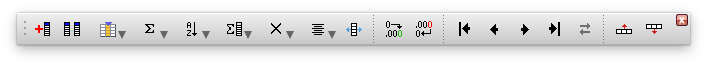Table 4-30. Column toolbar commands.

IconCommandKeyDescription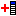Table -> Add ColumnAlt-CAdds a new column to the selected table.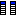Table -> Duplicate ColumnsCtrl-DDuplicates the selected columns in the table. The new columns are appended to the table and have the same properties and values as the source columns, except the name.Table -> Insert Column Inserts a new column to the left of the selected column(s) in the active table. This action is enabled only if there is at least one column selected in the active table.Table -> Insert Row Inserts a new row above the selected row in the active table. This operation works only if there is at least one row selected in the active table.Table -> Set Column Values...Alt-QUsed for functional assignment of values in selected column.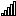Table -> Fill Column With -> Row Numbers Fills each row in the selected column with it's corresponding row number.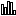Table -> Fill Column With -> Random Numbers Fills each row in the selected column with a random value.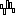Table -> Fill Column With -> Normal Random Numbers Fills each row in the selected column with a normally distributed random value.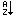Analysis -> Sort Column -> Ascending Sorts selected columns separately in ascending order.Analysis -> Sort Column -> Descending Sorts selected columns separately in descending order.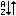Analysis -> Sort Table Sorts all columns in the selected table into ascending or descending order.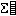Analysis -> Statistics on Columns Computes statistical parameters on selected columns.Analysis -> Statistics on Rows Computes statistical parameters on the selected rows.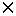Table -> Set Column As -> X Define the selected column as abscissae for plotting.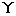Table -> Set Column As -> Y Define the selected column as an ordinate for plotting.Table -> Set Column As -> Z For 3D plots, Z columns will be used as height values.Table -> Set Column As -> Y error Define the selected column for use as error bars for Y-values.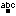Table -> Set Column As -> label Define the selected column as containing labels.Table -> Set Column As -> Disregard This command removes any plot role from selected columns.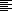Table -> Alignment -> Alignment -> Left The texts in the selected columns are aligned with the left edge of the columns.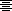Table -> Alignment -> Alignment -> Center The texts in the selected columns are centered horizontally in the available space.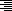Table -> Alignment -> Alignment -> Right The texts in the selected columns are aligned with the right edge of the columns.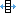Table -> Adjust Column Width Sets an optimal width on the selected columns.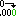Table -> Increase Precision Increases the number of digits displayed in the selected columns.Table -> Decrease Precision Decreases the number of digits displayed in the selected columns.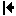Table -> Move to First Moves the selected column to the beginning of the table.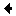Table -> Move Left Moves the selected column to the left.Table -> Move Right Moves the selected column to the right.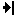Table -> Move to Last Moves the selected column to the end of the table.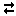Table -> Swap columns Swap the selected columns.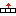Table -> Move Row -> UP Moves selected row UP one slot in table.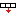Table -> Move Row -> DOWN Moves selected row DOWN one slot in table.